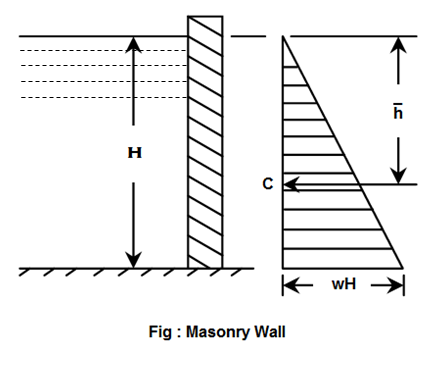•http://facebook.com/
•https://www.google.com/accounts/o8/id
•https://me.yahoo.com

# Masonry Walls

Water pressure on masonry walls

## Overview

Consider a vertical masonry wall having water on one of its sides as shown in figure. Now consider a unit length of the wall. We know that the water pressure will act perpendicular to the wall. A little consideration will show, that the intensity of pressure, at the water level, will be zero, and will increase by a straight line law to at the bottom as shown in figure. Thus the pressure diagram will be a triangle.The total pressure on the wall will be the area of the triangle, i.e.,

This pressure will act through the center of gravity of the pressure diagram.

Let, = Depth of the center of pressure from the water surface.

We know that the c.g. of triangle is at a height of from the base, where is the height of the triangle. Therefore depth of center of pressure from the water surface,

Thus the pressure of water on a vertical wall will act through a point at a distance from the bottom, where is the depth of water.

Example:
[metric]
##### Example - Water pressure on masonry walls
Problem
One of the walls of a swimming pool contains 4m deep water. Determine the total pressure on the wall, if it is 10m wide.
Workings
Given,

• Depth of water, H = 4m
• Width of wall = 10m

We know that pressure on the wall per meter length

and total pressure on the wall,
Solution
Total pressure on the wall = 784.8 KN